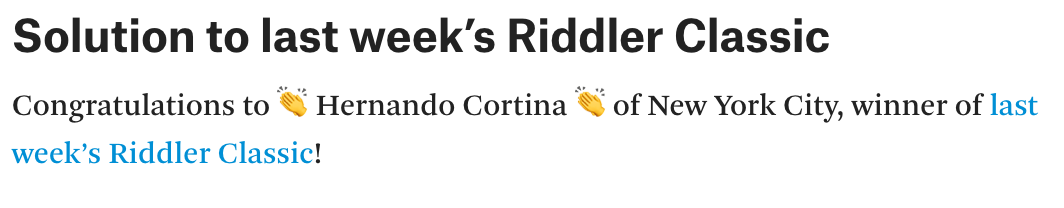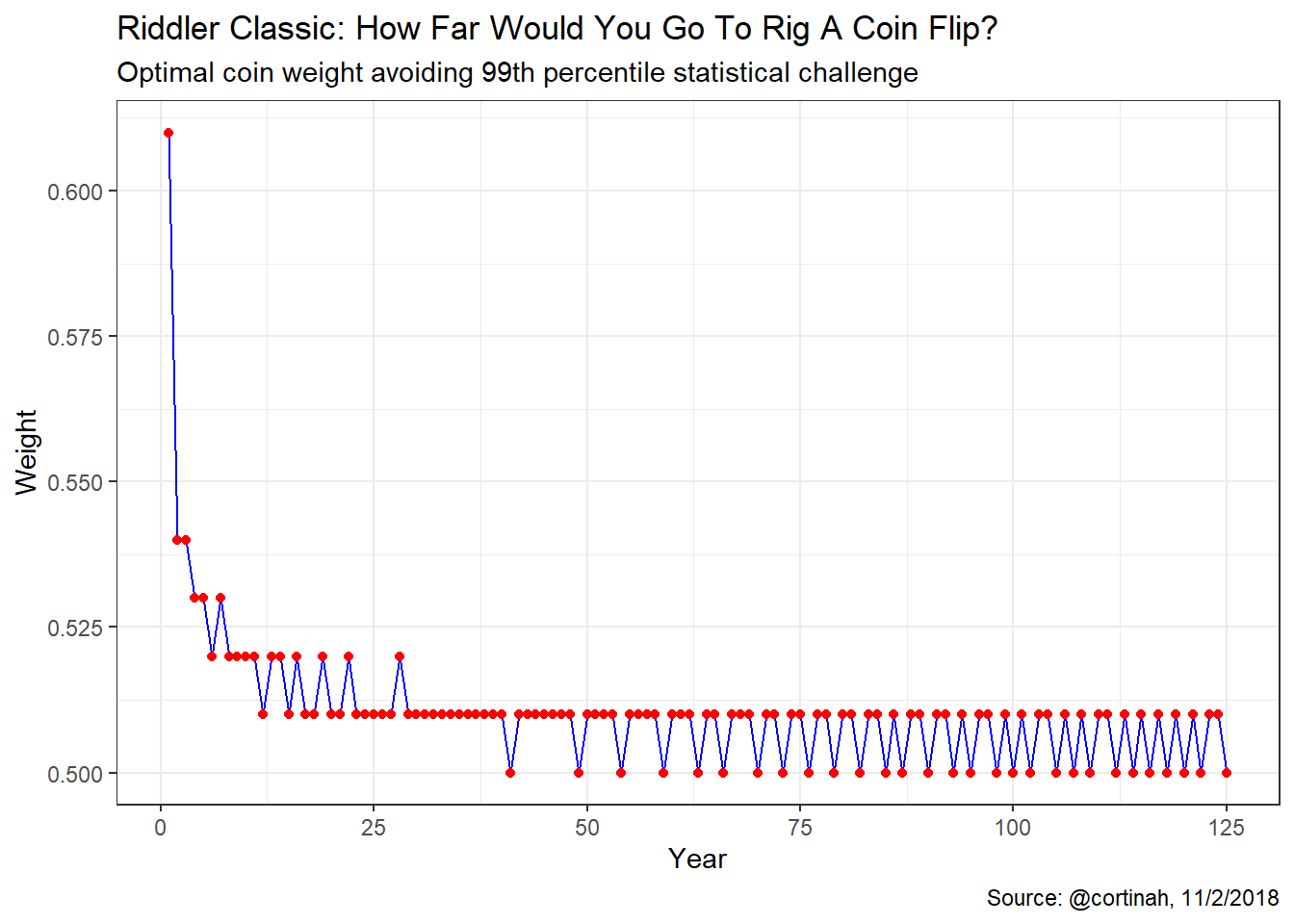# Riddler Classic: How Far Would You Go To Rig A Coin Flip?

## An Election Probability Riddler Classic, Just in Time for November 6

This week’s FiveThirtyEight Riddler Classic was about winning elections, fair and square, or by any means necessary… In this case, it’s Programmers versus Theorists, and elections are decided by flipping coins for each of one hundred Senate seats. As an analyst for Riddler Nation’s wildly successful data-driven political blog, OneHundred, these are the questions we are confronted with today:

### UPDATE (November 9): 😀 🥇 🍾 🎉 Hooray! My solution was chosen by @ollie as last week’s winner. Thank you very much Ollie for the kind write-up. 😀 🥇 🍾 🎉## Question 1: What is the probability that one party will win a simple majority?

### Need 51 or more Senate seats for a simple majority

$P=\sum_{k=51}^{k=100}\binom{100}{k}p^{k}(1-p)^{100-k}\;\;\;\;\;\;\;\;\;\;\;\;\;(1)$

$With\; a\; fair\; coin:\;\;p = \frac{1}{2}$ $P= \left ( \frac{1}{2} \right )^{100}\;\sum_{k=51}^{k=100}\binom{100}{k}$

senators <- 51:100
sum(choose(100, senators)) * (1/2)^100

 0.4602

### Need 60 or more Senate seats for a supermajority

senators <- 60:100
sum(choose(100, senators)) * (1/2)^100

 0.02844

## Question 2: what weighting would give them a 50% chance of winning a 60-seat supermajority?

From equation (1), must solve for p such that: $\frac{1}{2}=\sum_{k=60}^{k=100}\binom{100}{k}p^{k}(1-p)^{100-k}$ Find the root in (0, 1) of: $\sum_{k=60}^{k=100}\binom{100}{k}p^{k}(1-p)^{100-k}-\frac{1}{2}=0$

senators <- 60:100
weighting <- function(x) sum(choose(100, senators) * (x^senators) * (1-x)^(100-senators)) - 0.5
result <- uniroot(weighting, c(0,1))
result$root  0.5947 ### How often can the Programmers cheat before the Theorists can prove there’s at least a 99% chance that the coin wasn’t fair? Each election consists of a binomial distribution with n=100 and under the null hypothesis that the coin is fair p = 1/2. Accordingly, we can look at the 99% quantile of this binomial until the number of Programmers winning falls outside the 99% quantile. With one election (n=100) the 99th-percentile is 62, so a Programmer supermajority is more than 1% likely. With two elections (n=200) the 99th-percentile is 116, so the likelihood of the Programmers winning more than 60 seats in each election (120 total) falls outside the 99th-percentile. Thus, the theorists can prove the Programmers cheat after two election cycles. qbinom(c(0, 0.99), size=100, prob=0.5)  0 62 qbinom(c(0, 0.99), size=200, prob=0.5)  0 116 ## Question 3: If the Programmers decide to cheat by weighting the coin permanently for the next 100 elections, how heavily can they weight it and escape a 99% challenge by the Theorists? Over 100 years there will be 10,000 separate coin flips. The 99th-percentile of this binomial distribution is 5,116. This implies they can cheat by giving themselves a probability p up to 0.5116 qbinom(c(0, 0.99), size=10000, prob=0.5)  0 5116 ### How many 60-seat supermajorities can they expect to win over this 100-year period? We use equation (1) to calculate the probability of winning a supermajority in a given election. This is approximately 4.7%. Accordingly, the Programmers can expect to win 4-5 supermajorities over a century. p <- 0.5116 senators <- 60:100 sum(choose(100, senators) * (p^senators) * (1-p)^(100-senators))  0.04723 100 * sum(choose(100, senators) * (p^senators) * (1-p)^(100-senators))  4.723 ## Question 4: What is the optimal cheating strategy for the Programmers? We calculate for each year the cumulative 99th percentile of coin flips the Programmers can win while remaining “innocent” before the Statistical Court. We subtract one from that amount and determine the required coin weight to produce those expected wins. optimal <- as.data.frame(matrix(NA,nrow=125,ncol=5)) colnames(optimal) <- c('Year','quantile','opt','diff','Weight') optimal$Year <- 1:125
optimal$quantile <- qbinom(0.99, size=100*optimal$Year, prob=0.5)
optimal$opt <- optimal$quantile-1
optimal$diff[2:125] <- diff(optimal$opt); optimal$diff <- 61 optimal$Weight <- optimal\$diff/100We notice an interesting oscillatory pattern where after cravenly cheating for over 30 years, the Programmers’ coin needs to be fair in a growing proportion of elections over time. When they have enough “buffer”, the Programmers can cheat for an election, or two, before returning to a fair coin.

## Question 5: Does changing the coin weight on a seat-by-seat basis help improve the Programmers’ odds in any of these scenarios?

No, it wouldn’t because the Theorists are checking the election results on an election-by-election basis, based on the number of seats won by the Programmers, which is an integer value that must be within the 99th percentile of a binomial distribution. Setting different win probabilities by seat does not change the check of the overall election.